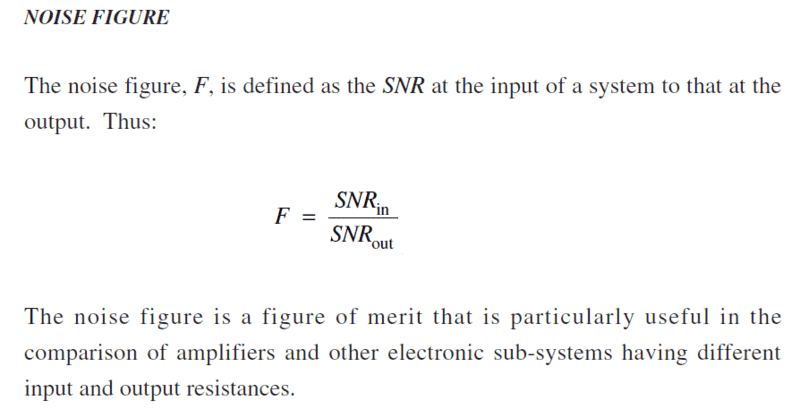# Signal to Noise Ratio Help

• Comp Sci
• Jason-Li

#### Jason-Li

Homework Statement
(a) Determine the voltage required on the input of the amplifier to give
the maximum output of 85 dBμV.
(b) If the signal level from the aerial is 5 dBmV and the input noise level
is 20 dBμV, calculate the signal-to-noise ratio on the output of the
amplifier.

Values:
Bandwidth 40–862 MHz
Gain 20 dB
Noise Figure 6 dB
Max. Output 85 dBV
Input Impedance 75Ω
Output Impedance 75Ω
Relevant Equations
SNR=20Log(Vs/Vn)
SNR=10Log(Ps/Pn)
So pretty confident I understand part (a) however for part (b) I'm not sure if I have carried it out correctly if someone could give me a pointer?

(b)
5dBmV Input as a voltage:
5=20Log(V/1mV)
V=105/10
V=1.77827941mV

Then the noise level is 20dbμV so changing to a voltage:
20=20*Log(V/1μV)
V=1020/20
V=10μV

Then I can find the SNR of the input using:
SNRIn=20*Log(Vp/Vn)
SNRIn=20*Log((1.77827941*10-3)/(10*10-6))
SNRIn=45dB

Then as I have the Noise figure from the data as F=6dB I can do the following:
F=SNRIn/SNROut
SNROut=SNRIn/F
SNROut=45*6
SNROut=270dB

This seems far too high? I have found websites that say the formula is actually F = SNRIn-SNROut however the learning materials say:I think you are correct as far as part (b). Then you know the SNR at the input, and the SNR at the output will be 6dB worse. So SNRout = 45 - 6 = 39dB.

•Jason-Li
I think you are correct as far as part (b). Then you know the SNR at the input, and the SNR at the output will be 6dB worse. So SNRout = 45 - 6 = 39dB.
Hi thanks for the reply and assurance, do you have any idea why my learning materials is citing the noise figure equation incorrectly?

Yes, the formula for F is not stated in decibels. To divide decibel quantities we subtract, not divide.

•Merlin3189 and Jason-Li
Homework Statement:: (a) Determine the voltage required on the input of the amplifier to give
the maximum output of 85 dBμV.
(b) If the signal level from the aerial is 5 dBmV and the input noise level
is 20 dBμV, calculate the signal-to-noise ratio on the output of the
amplifier.

Values:
Bandwidth 40–862 MHz
Gain 20 dB
Noise Figure 6 dB
Max. Output 85 dBV
Input Impedance 75Ω
Output Impedance 75Ω
Relevant Equations:: SNR=20Log(Vs/Vn)
SNR=10Log(Ps/Pn)

So pretty confident I understand part (a) however for part (b) I'm not sure if I have carried it out correctly if someone could give me a pointer?

(b)
5dBmV Input as a voltage:
5=20Log(V/1mV)
V=105/10
V=1.77827941mV

Then the noise level is 20dbμV so changing to a voltage:
20=20*Log(V/1μV)
V=1020/20
V=10μV

Then I can find the SNR of the input using:
SNRIn=20*Log(Vp/Vn)
SNRIn=20*Log((1.77827941*10-3)/(10*10-6))
SNRIn=45dB

Then as I have the Noise figure from the data as F=6dB I can do the following:
F=SNRIn/SNROut
SNROut=SNRIn/F
SNROut=45*6
SNROut=270dB

This seems far too high? I have found websites that say the formula is actually F = SNRIn-SNROut however the learning materials say:

View attachment 290575

Hi, I am struggling with part A to this question, could you assist? I am not sure where to start.

In Part (a) the output of the amplifier is 85 dBuV and the gain of the amplifier is 20 dB. How many dBuV aat the input?

Do I need to convert dB into Voltage for both gain and Vo and then just use a transposed ratio for gain to calculate Vi?

No you can stay in dB entirely. Do not over think this one!

With gain just being a ratio, is it as simple as G = output / input... transformed giving 85 / 20 = Vi (however needing to subtract rather than divide) 85 - 20 = Vi?

Last edited:
When we multiply or divide quantities using decibels we simply add or subtract. So for instance, if I have a signal of 10 dBV and I amplify it by 6 dB I end up with a signal of 10 + 6 = 16 dBV.
The whole point of decibels is to make gains and losses simple to work out without the need to carry out multiplication or division.
It is only if voltages or powers are actually added or subtracted that we have to come out of the decibel system.